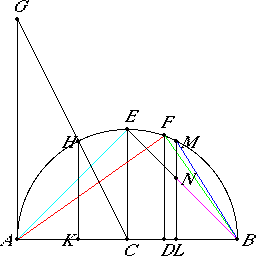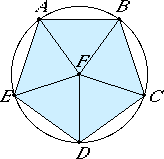# Proposition 18

To set out the sides of the five figures and compare them with one another.
I.11

Set out AB the diameter of the given sphere, and cut it at C so that AC equals CB, and at D so that AD is double DB. Describe the semicircle AEB on AB, draw CE and DF from C and D at right angles to AB, and join AF, FB, and EB.

Then, since AD is double DB, therefore AB is triple BD. In conversion, therefore, BA is one and a half times AD.But BA is to AD as the square on BA is to the square on AF, for the triangle AFB is equiangular with the triangle AFD. Therefore the square on BA is one and a half times the square on AF.

XIII.13

But the square on the diameter of the sphere is also one and a half times the square on the side of the pyramid. And AB is the diameter of the sphere, therefore AF equals the side of the pyramid.

Again, since AD is double DB, therefore AB is triple BD. But AB is to BD as the square on AB to the square on BF, therefore the square on AB is triple the square on BF.

XIII.15

But the square on the diameter of the sphere is also triple the square on the side of the cube. And AB is the diameter of the sphere, therefore BF is the side of the cube.

And, since AC equals CB, therefore AB is double BC. But AB is to BC as the square on AB to the square on BE, therefore the square on AB is double the square on BE.

XIII.14

But the square on the diameter of the sphere is also double the square on the side of the octahedron. And AB is the diameter of the given sphere, therefore BE is the side of the octahedron.

Next, draw AG from the point A at right angles to the straight line AB, make AG equal to AB, join GC, and draw HK from H perpendicular to AB.

Then, since GA is double AC, for GA equals AB and GA is to AC as HK is to KC, therefore HK is also double KC.

Therefore the square on HK is quadruple the square on KC, therefore the sum of the squares on HK and KC, that is, the square on HC, is five times the square on KC.

But HC equals CB, therefore the square on BC is five times the square on CK. And, since AB is double CB, and, in them, AD is double DB, therefore the remainder BD is double the remainder DC.

Therefore BC is triple CD, therefore the square on BC is nine times the square on CD. But the square on BC is five times the square on CK, therefore the square on CK is greater than the square on CD. Therefore CK is greater than CD.

Make CL equal to CK, draw LM from L at right angles to AB, and join MB.

Now, since the square on BC is five times the square on CK, and AB is double BC, and KL is double CK, therefore the square on AB is five times the square on KL. But the square on the diameter of the sphere is also five times the square on the radius of the circle from which the icosahedron has been described. And AB is the diameter of the sphere, therefore KL is the radius of the circle from which the icosahedron has been described. Therefore KL is a side of the hexagon in the said circle.

XIII.16,Cor.

And, since the diameter of the sphere is made up of the side of the hexagon and two of the sides of the decagon inscribed in the same circle, and AB is the diameter of the sphere, while KL is a side of the hexagon, and AK equals LB, therefore each of the straight lines AK and LB is a side of the decagon inscribed in the circle from which the icosahedron has been described.

XIII.10

And, since LB belongs to a decagon, and ML to a hexagon, for ML equals KL, since it also equals HK being the same distance from the center and each of the straight lines HK and KL is double KC, therefore MB belongs to a pentagon.

XIII.16

But the side of the pentagon is the side of the icosahedron, therefore MB belongs to the icosahedron.

XIII.17,Cor.

Now, since FB is a side of the cube, cut it in extreme and mean ratio at N, and let NB be the greater segment. Therefore NB is a side of the dodecahedron.

And, since the square on the diameter of the sphere was proved to be one and a half times the square on the side AF of the pyramid, double the square on the side BE of the octahedron and triple the side FB of the cube, therefore, of parts of which the square on the diameter of the sphere contains six, the square on the side of the pyramid contains four, the square on the side of the octahedron three, and the square on the side of the cube two.

Therefore the square on the side of the pyramid is four-thirds of the square on the side of the octahedron, and double the square on the side of the cube, and the square on the side of the octahedron is one and a half times the square on the side of the cube.

The said sides, therefore, of the three figures, I mean the pyramid, the octahedron and the cube, are to one another in rational ratios.

But the remaining two, I mean the side of the icosahedron and the side of the dodecahedron, are not in rational ratios either to one another or to the aforesaid sides, for they are irrational, the one being minor and the other an apotome.

That the side MB of the icosahedron is greater than the side NB of the dodecahedron we can prove thus.

Since the triangle FDB is equiangular with the triangle FAB, proportionally DB is to BF as BF is to BA.

And, since the three straight lines are proportional, the first is to the third as the square on the first is to the square on the second, therefore DB is to BA as the square on DB is to the square on BF. Therefore, inversely AB is to BD as the square on FB is to the square on BD.

But AB is triple BD, therefore the square on FB is triple the square on BD.

But the square on AD is also quadruple the square on DB, for AD is double DB, therefore the square on AD is greater than the square on FB. Therefore AD is greater than FB. Therefore AL is by far greater than FB.

XIII.9

And, when AL is cut in extreme and mean ratio, KL is the greater segment, for LK belongs to a hexagon, and KA to a decagon, and, when FB is cut in extreme and mean ratio, NB is the greater segment, therefore KL is greater than NB.

But KL equals LM, therefore LM is greater than NB.

Therefore MB, which is a side of the icosahedron, is by far greater than NB which is a side of the dodecahedron.

Q.E.F.

# Remark

I say next that no other figure, besides the said five figures, can be constructed which is contained by equilateral and equiangular figures equal to one another.

For a solid angle cannot be constructed with two triangles, or indeed planes.

XI.21

With three triangles the angle of the pyramid is constructed, with four the angle of the octahedron, and with five the angle of the icosahedron, but a solid angle cannot be formed by six equilateral and equiangular triangles placed together at one point, for, the angle of the equilateral triangle being two-thirds of a right angle, the six would be equal to four right angles, which is impossible, for any solid angle is contained by angles less than four right angles.

For the same reason, neither can a solid angle be constructed by more than six plane angles.

By three squares the angle of the cube is contained, but by four it is impossible for a solid angle to be contained, for they would again be four right angles.

By three equilateral and equiangular pentagons the angle of the dodecahedron is contained, but by four such it is impossible for any solid angle to be contained, for, the angle of the equilateral pentagon being a right angle and a fifth, the four angles would be greater than four right angles, which is impossible.

Neither again will a solid angle be contained by other polygonal figures by reason of the same absurdity.

Q.E.D.

# Lemma

But that the angle of the equilateral and equiangular pentagon is a right angle and a fifth we must prove thus.IV.14

Let ABCDE be an equilateral and equiangular pentagon. Circumscribe the circle ABCDE about it, take its center F, and join FA, FB, FC, FD, and FE.

Therefore they bisect the angles of the pentagon at A, B, C, D, and E. And, since the angles at F equal four right angles and are equal, therefore one of them, as the angle AFB, is one right angle less a fifth. Therefore the remaining angles FAB and ABF consist of one right angle and a fifth.

But the angle FAB equals the angle FBC, therefore the whole angle ABC of the pentagon consists of one right angle and a fifth.

Q.E.D.

## Guide

#### Summary of the regular polyhedra

In the following table, d is the diameter of the sphere in which each regular polygon is inscribed while s is the side of the polygon. Then d2/s2 is the ratio of the square of the diameter to the square of the side.

Polyhedron construction d2/s2
tetrahedron XIII.13 3/2
octahedron XIII.14 2
cube XIII.15 3
icosahedron XIII.16 (2√5)/(√5–1)
dodecahedron XIII.17 (3–√5)/6